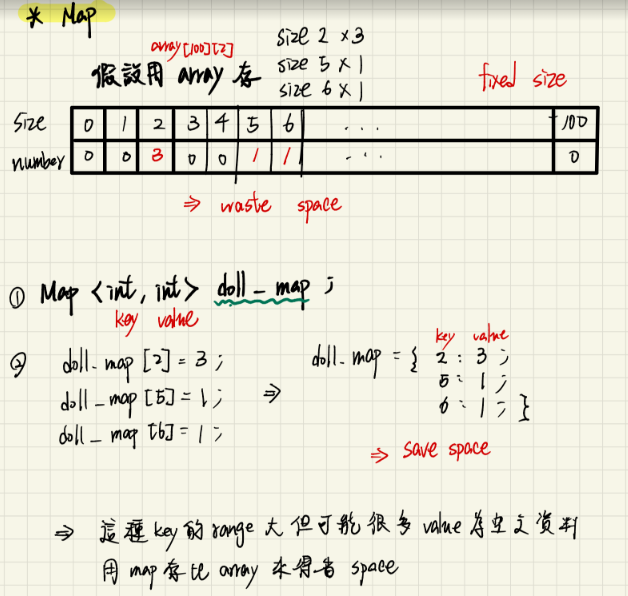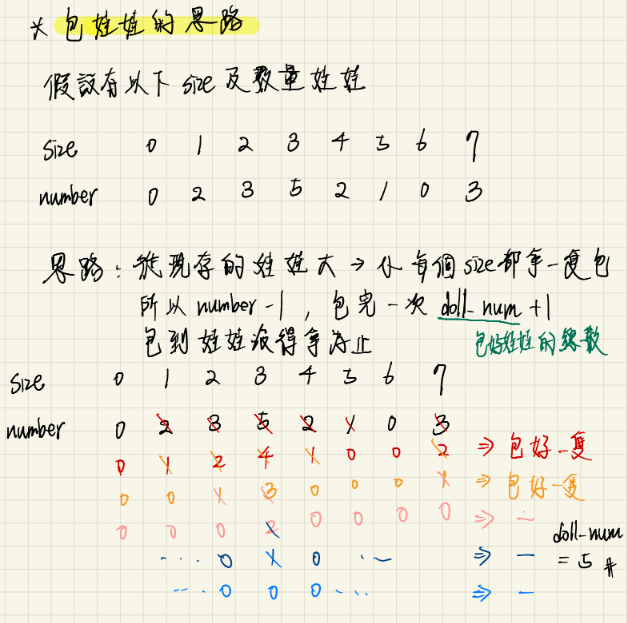8
1 5 3 7 2 2 2 1

# output自此始，但不包括此行

3

map<> get_input(map<> doll_map){

``````//
``````

}

void calculate_doll(map<>& doll_map){

``````//
``````

}

1. 不會有第一行輸入與第二行輸入個數不相符之測資。
2. 個數及大小皆為大於0且小於等於100的正整數。

# 完整程式碼

``````#include<iostream>
//1. import the lib
#include<map>
using namespace std;

//3 把娃娃以size比對數量建立map
map<int,int> get_input(map<int,int> doll_map,int num){
for(int i=0;i<num;i++){
int size;
//輸入size的娃娃數量+1
cin >> size;
doll_map[size]++;
}
//返回建立好的map
return doll_map;
}

//4.
void calculate_doll(map<int,int>& doll_map){
int dollNum=0;          //計算包起來的總娃娃數
bool hasDoll = true;    //表示還有娃娃沒有被包起來

//開始包娃娃,包到沒有為止 (包娃娃-->各還有現存的size其數量都-1 , 全部size數量都0表示包完了)
while(hasDoll){
//一開始不知道有沒有娃娃還沒包 -->先假設沒有
hasDoll=false;
for(int i=0;i<=100;i++){
//表示有包到娃娃 hasDoll = true
if(doll_map[i]>0){
hasDoll = true;
doll_map[i]--;
}
}
//有包到娃娃,dollNum+1
if(hasDoll) dollNum++;
}

cout << dollNum << endl;
}

int main(){

//2.輸入的娃娃數量
int num;
cin >> num;

//3.建立map來輸入每個大小娃娃的數量
map<int,int> doll_map;
doll_map = get_input(doll_map,num);

//4.計算最後娃娃數量
calculate_doll(doll_map);

return 0;
}
``````

# 解題觀念及思路